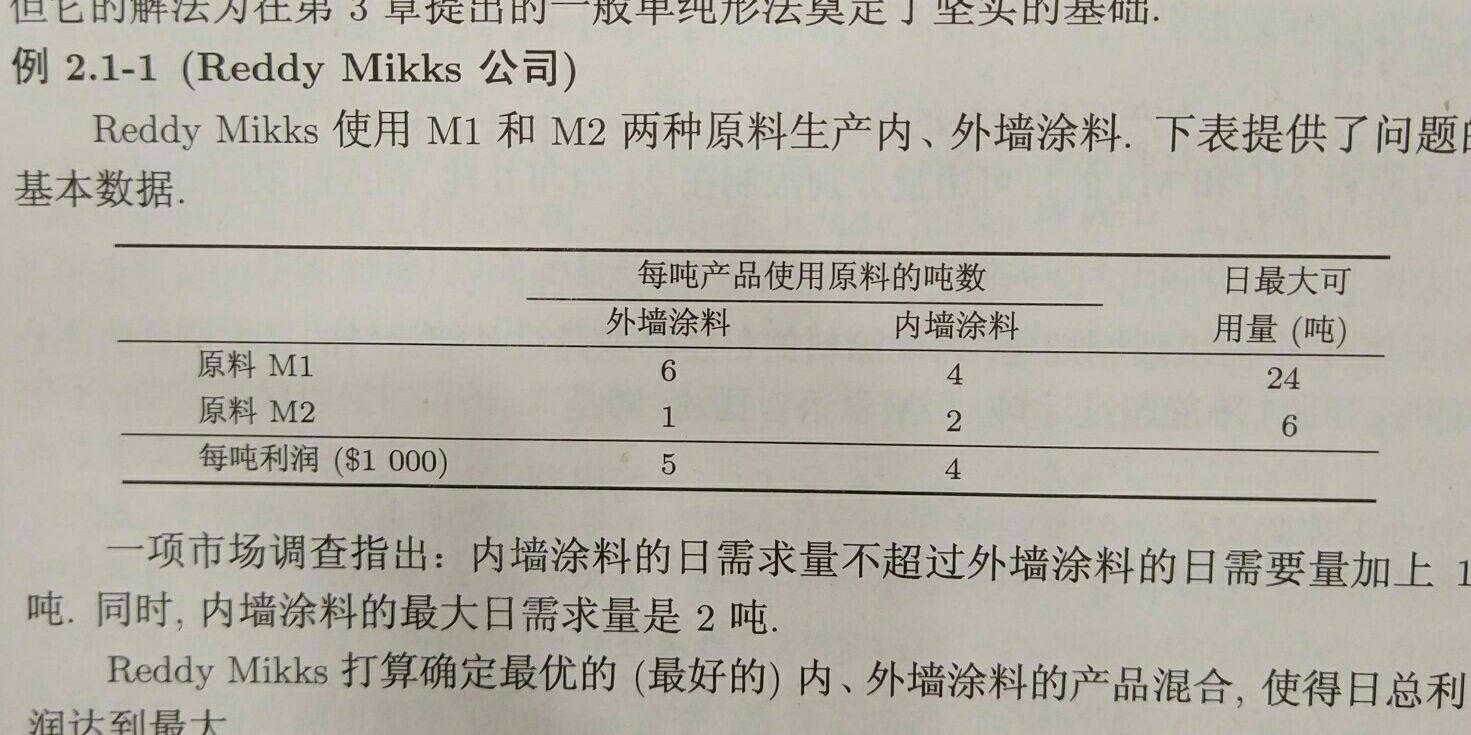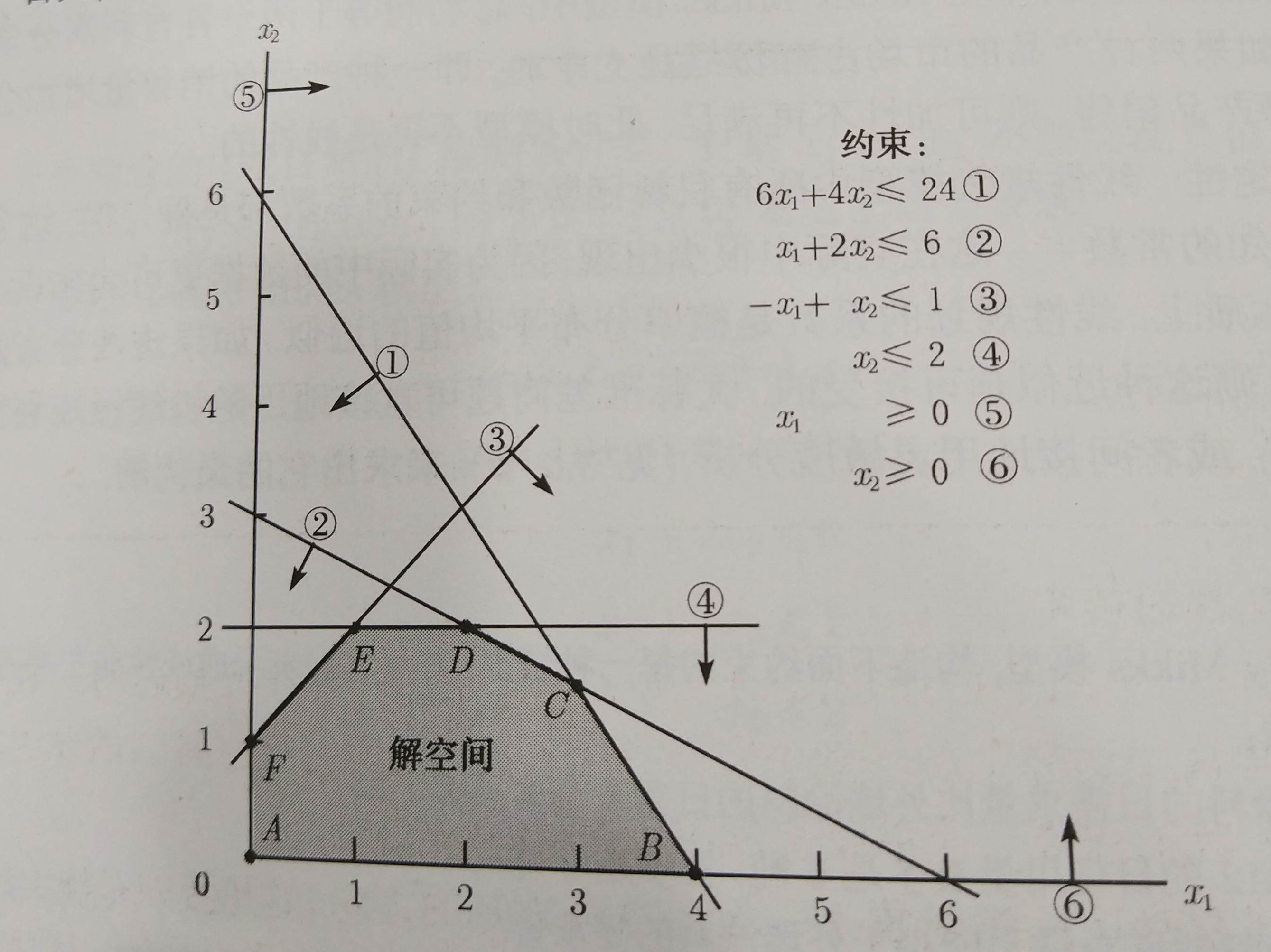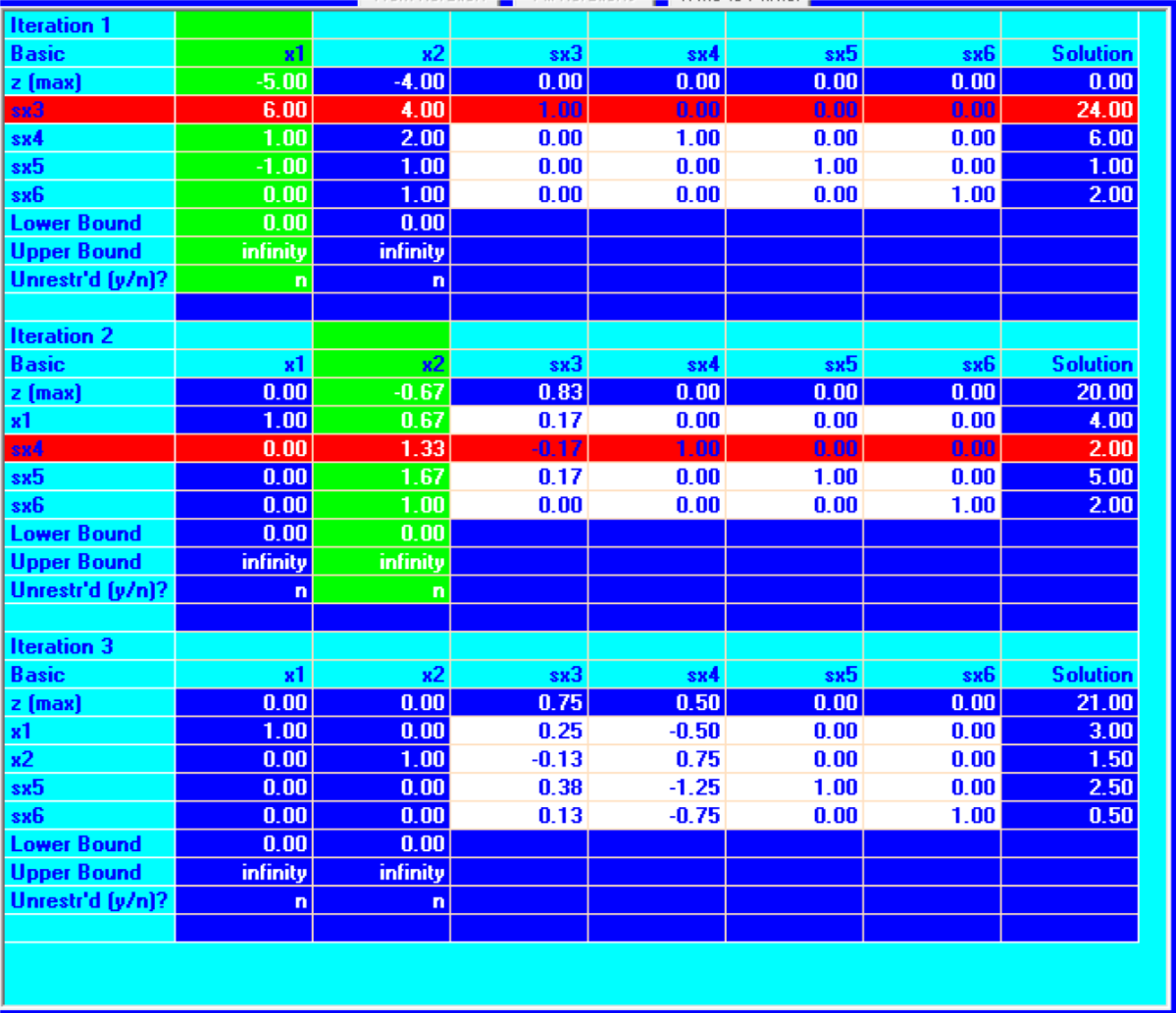10 篇文章 0 订阅

• 几何表示
• 标准型的代数解法
• 其他情形与标准型的互换
• 退化情形

# 几何表示max zs.t 6x1+4x2x1+2x2x1+x2x2xi=5x1+4x2246120# 代数形式

max zs.t i=1najixixi=i=1ncixibj(j=1,2,,m)0,i=1,2,3,,n

## 单纯形(simplex) 算法

6x1+4x224 $6x_1+4x_2\le24$

6x1+4x2+x3=24 $6x_1+4x_2+x_3=24$

max zs.t 6x1+4x2+x3x1+2x2+x4x1+x2+x5x2+x6xi=5x1+4x2=24=6=1=20

x3=24(6x1+4x2) $x_3=24-(6x_1+4x_2)$

1. #basic var （基本变量的数目）=函数限制的数目。
2. # non-basic var = #var - #basic var = 线性规划系统的自由度
3. non-basic var =0
4. 如果 基本变量满足非负限制，则基解称为 基本可行解(basic feasible solution BF),我们后面需要关注的就是这个基本可行解BF，这对应的是原始形式中的CPF 的扩充。(非松弛变量为0是初始解)

1. 初始化，解除限制，引入松弛变量，将目标函数写成 (z(sumni=1cixi)=0) $(z-(sum_{i=1}^n c_ix_i)=0)$ 记作第0行
2. 最优性测试，选出第0行中系数最小的变量（负的最多），判断系数是否小于0,若是结束输出最优解，否则进入迭代,称选中变量为进基变量(变量不为0，成为基本变量)
3. 进入迭代,进基变量对应的列称为轴列,pivot col,进行最优比率测试，判断，进基变量能最大增加多少. 对于轴列中系数大于0的列，用等式右边值除以系数得到每个等式的比率.,最小的一个作为瓶颈行，称为轴行(pivot row)
4. 用轴行进行高斯肖元，回到第二步.# 非标准型与标准型的转化

aixiaixibb

## 负的右值限制

aixib,b>0 $\sum a_i*x_i \le -b,b>0$,乘一个负号变为 aixib $\sum -a_i*x_i \ge b$

## 大于等于限制

0.6x1+0.4x26 $0.6x_1 + 0.4x_2 \ge 6$, 引入 x3>0 $x_3>0$,转化为等式

0.6x1+0.4x2x3=6 $0.6x_1 + 0.4x_2 -x_3 = 6$,这是等价的

## 无界限制

xi(inf,inf) $x_i\in (-inf,inf)$,
xi=x+ixi,x+i,xi>0 $x_i = x_i^{+}-x_i^{-},x_i^{+},x_i^{-}>0$,需要注意的是若有很多变量均是无界限制，可以只取一个变量代替 xi $x_i^{-}$，而不必对每个无界变量都引入两个变量.

09-3010-15
04-19
03-17172
11-201663
03-161017
09-221万+
01-242874
07-121万+
02-01754
04-24
12-131万+
12-158182点击重新获取扫码支付余额充值